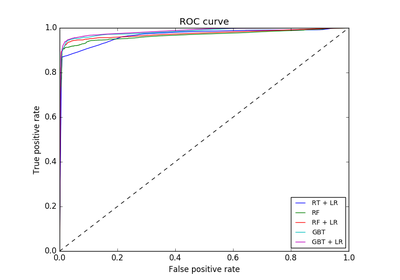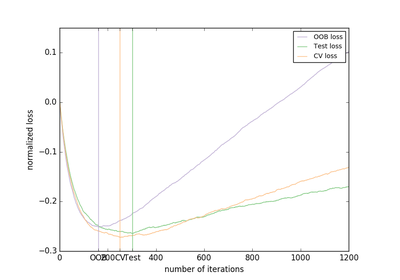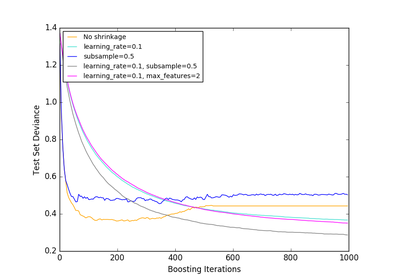# 3.2.4.3.5. `sklearn.ensemble`.GradientBoostingClassifier¶

class `sklearn.ensemble.``GradientBoostingClassifier`(loss='deviance', learning_rate=0.1, n_estimators=100, subsample=1.0, criterion='friedman_mse', min_samples_split=2, min_samples_leaf=1, min_weight_fraction_leaf=0.0, max_depth=3, min_impurity_split=1e-07, init=None, random_state=None, max_features=None, verbose=0, max_leaf_nodes=None, warm_start=False, presort='auto')[source]

GB builds an additive model in a forward stage-wise fashion; it allows for the optimization of arbitrary differentiable loss functions. In each stage `n_classes_` regression trees are fit on the negative gradient of the binomial or multinomial deviance loss function. Binary classification is a special case where only a single regression tree is induced.

Read more in the User Guide.

Parameters: loss : {‘deviance’, ‘exponential’}, optional (default=’deviance’) loss function to be optimized. ‘deviance’ refers to deviance (= logistic regression) for classification with probabilistic outputs. For loss ‘exponential’ gradient boosting recovers the AdaBoost algorithm. learning_rate : float, optional (default=0.1) learning rate shrinks the contribution of each tree by learning_rate. There is a trade-off between learning_rate and n_estimators. n_estimators : int (default=100) The number of boosting stages to perform. Gradient boosting is fairly robust to over-fitting so a large number usually results in better performance. max_depth : integer, optional (default=3) maximum depth of the individual regression estimators. The maximum depth limits the number of nodes in the tree. Tune this parameter for best performance; the best value depends on the interaction of the input variables. criterion : string, optional (default=”friedman_mse”) The function to measure the quality of a split. Supported criteria are “friedman_mse” for the mean squared error with improvement score by Friedman, “mse” for mean squared error, and “mae” for the mean absolute error. The default value of “friedman_mse” is generally the best as it can provide a better approximation in some cases. New in version 0.18. min_samples_split : int, float, optional (default=2) The minimum number of samples required to split an internal node: If int, then consider min_samples_split as the minimum number. If float, then min_samples_split is a percentage and ceil(min_samples_split * n_samples) are the minimum number of samples for each split. Changed in version 0.18: Added float values for percentages. min_samples_leaf : int, float, optional (default=1) The minimum number of samples required to be at a leaf node: If int, then consider min_samples_leaf as the minimum number. If float, then min_samples_leaf is a percentage and ceil(min_samples_leaf * n_samples) are the minimum number of samples for each node. Changed in version 0.18: Added float values for percentages. min_weight_fraction_leaf : float, optional (default=0.) The minimum weighted fraction of the sum total of weights (of all the input samples) required to be at a leaf node. Samples have equal weight when sample_weight is not provided. subsample : float, optional (default=1.0) The fraction of samples to be used for fitting the individual base learners. If smaller than 1.0 this results in Stochastic Gradient Boosting. subsample interacts with the parameter n_estimators. Choosing subsample < 1.0 leads to a reduction of variance and an increase in bias. max_features : int, float, string or None, optional (default=None) The number of features to consider when looking for the best split: If int, then consider max_features features at each split. If float, then max_features is a percentage and int(max_features * n_features) features are considered at each split. If “auto”, then max_features=sqrt(n_features). If “sqrt”, then max_features=sqrt(n_features). If “log2”, then max_features=log2(n_features). If None, then max_features=n_features. Choosing max_features < n_features leads to a reduction of variance and an increase in bias. Note: the search for a split does not stop until at least one valid partition of the node samples is found, even if it requires to effectively inspect more than `max_features` features. max_leaf_nodes : int or None, optional (default=None) Grow trees with `max_leaf_nodes` in best-first fashion. Best nodes are defined as relative reduction in impurity. If None then unlimited number of leaf nodes. min_impurity_split : float, optional (default=1e-7) Threshold for early stopping in tree growth. A node will split if its impurity is above the threshold, otherwise it is a leaf. New in version 0.18. init : BaseEstimator, None, optional (default=None) An estimator object that is used to compute the initial predictions. `init` has to provide `fit` and `predict`. If None it uses `loss.init_estimator`. verbose : int, default: 0 Enable verbose output. If 1 then it prints progress and performance once in a while (the more trees the lower the frequency). If greater than 1 then it prints progress and performance for every tree. warm_start : bool, default: False When set to `True`, reuse the solution of the previous call to fit and add more estimators to the ensemble, otherwise, just erase the previous solution. random_state : int, RandomState instance or None, optional (default=None) If int, random_state is the seed used by the random number generator; If RandomState instance, random_state is the random number generator; If None, the random number generator is the RandomState instance used by np.random. presort : bool or ‘auto’, optional (default=’auto’) Whether to presort the data to speed up the finding of best splits in fitting. Auto mode by default will use presorting on dense data and default to normal sorting on sparse data. Setting presort to true on sparse data will raise an error. New in version 0.17: presort parameter. feature_importances_ : array, shape = [n_features] The feature importances (the higher, the more important the feature). oob_improvement_ : array, shape = [n_estimators] The improvement in loss (= deviance) on the out-of-bag samples relative to the previous iteration. `oob_improvement_` is the improvement in loss of the first stage over the `init` estimator. train_score_ : array, shape = [n_estimators] The i-th score `train_score_[i]` is the deviance (= loss) of the model at iteration `i` on the in-bag sample. If `subsample == 1` this is the deviance on the training data. loss_ : LossFunction The concrete `LossFunction` object. init : BaseEstimator The estimator that provides the initial predictions. Set via the `init` argument or `loss.init_estimator`. estimators_ : ndarray of DecisionTreeRegressor, shape = [n_estimators, `loss_.K`] The collection of fitted sub-estimators. `loss_.K` is 1 for binary classification, otherwise n_classes.

References

J. Friedman, Greedy Function Approximation: A Gradient Boosting Machine, The Annals of Statistics, Vol. 29, No. 5, 2001.

1. Friedman, Stochastic Gradient Boosting, 1999

T. Hastie, R. Tibshirani and J. Friedman. Elements of Statistical Learning Ed. 2, Springer, 2009.

Methods

 `apply`(X) Apply trees in the ensemble to X, return leaf indices. `decision_function`(X) Compute the decision function of `X`. `fit`(X, y[, sample_weight, monitor]) Fit the gradient boosting model. `fit_transform`(X[, y]) Fit to data, then transform it. `get_params`([deep]) Get parameters for this estimator. `predict`(X) Predict class for X. `predict_log_proba`(X) Predict class log-probabilities for X. `predict_proba`(X) Predict class probabilities for X. `score`(X, y[, sample_weight]) Returns the mean accuracy on the given test data and labels. `set_params`(\*\*params) Set the parameters of this estimator. `staged_decision_function`(X) Compute decision function of `X` for each iteration. `staged_predict`(X) Predict class at each stage for X. `staged_predict_proba`(X) Predict class probabilities at each stage for X. `transform`(\*args, \*\*kwargs) DEPRECATED: Support to use estimators as feature selectors will be removed in version 0.19.
`__init__`(loss='deviance', learning_rate=0.1, n_estimators=100, subsample=1.0, criterion='friedman_mse', min_samples_split=2, min_samples_leaf=1, min_weight_fraction_leaf=0.0, max_depth=3, min_impurity_split=1e-07, init=None, random_state=None, max_features=None, verbose=0, max_leaf_nodes=None, warm_start=False, presort='auto')[source]
`apply`(X)[source]

Apply trees in the ensemble to X, return leaf indices.

New in version 0.17.

Parameters: X : array-like or sparse matrix, shape = [n_samples, n_features] The input samples. Internally, its dtype will be converted to `dtype=np.float32`. If a sparse matrix is provided, it will be converted to a sparse `csr_matrix`. X_leaves : array_like, shape = [n_samples, n_estimators, n_classes] For each datapoint x in X and for each tree in the ensemble, return the index of the leaf x ends up in each estimator. In the case of binary classification n_classes is 1.
`decision_function`(X)[source]

Compute the decision function of `X`.

Parameters: X : array-like of shape = [n_samples, n_features] The input samples. score : array, shape = [n_samples, n_classes] or [n_samples] The decision function of the input samples. The order of the classes corresponds to that in the attribute classes_. Regression and binary classification produce an array of shape [n_samples].
`feature_importances_`
Return the feature importances (the higher, the more important the
feature).
Returns: feature_importances_ : array, shape = [n_features]
`fit`(X, y, sample_weight=None, monitor=None)[source]

Parameters: X : array-like, shape = [n_samples, n_features] Training vectors, where n_samples is the number of samples and n_features is the number of features. y : array-like, shape = [n_samples] Target values (integers in classification, real numbers in regression) For classification, labels must correspond to classes. sample_weight : array-like, shape = [n_samples] or None Sample weights. If None, then samples are equally weighted. Splits that would create child nodes with net zero or negative weight are ignored while searching for a split in each node. In the case of classification, splits are also ignored if they would result in any single class carrying a negative weight in either child node. monitor : callable, optional The monitor is called after each iteration with the current iteration, a reference to the estimator and the local variables of `_fit_stages` as keyword arguments ```callable(i, self, locals())```. If the callable returns `True` the fitting procedure is stopped. The monitor can be used for various things such as computing held-out estimates, early stopping, model introspect, and snapshoting. self : object Returns self.
`fit_transform`(X, y=None, **fit_params)[source]

Fit to data, then transform it.

Fits transformer to X and y with optional parameters fit_params and returns a transformed version of X.

Parameters: X : numpy array of shape [n_samples, n_features] Training set. y : numpy array of shape [n_samples] Target values. X_new : numpy array of shape [n_samples, n_features_new] Transformed array.
`get_params`(deep=True)[source]

Get parameters for this estimator.

Parameters: deep : boolean, optional If True, will return the parameters for this estimator and contained subobjects that are estimators. params : mapping of string to any Parameter names mapped to their values.
`predict`(X)[source]

Predict class for X.

Parameters: X : array-like of shape = [n_samples, n_features] The input samples. y: array of shape = [“n_samples] : The predicted values.
`predict_log_proba`(X)[source]

Predict class log-probabilities for X.

Parameters: X : array-like of shape = [n_samples, n_features] The input samples. p : array of shape = [n_samples] The class log-probabilities of the input samples. The order of the classes corresponds to that in the attribute classes_. AttributeError : If the `loss` does not support probabilities.
`predict_proba`(X)[source]

Predict class probabilities for X.

Parameters: X : array-like of shape = [n_samples, n_features] The input samples. p : array of shape = [n_samples] The class probabilities of the input samples. The order of the classes corresponds to that in the attribute classes_. AttributeError : If the `loss` does not support probabilities.
`score`(X, y, sample_weight=None)[source]

Returns the mean accuracy on the given test data and labels.

In multi-label classification, this is the subset accuracy which is a harsh metric since you require for each sample that each label set be correctly predicted.

Parameters: X : array-like, shape = (n_samples, n_features) Test samples. y : array-like, shape = (n_samples) or (n_samples, n_outputs) True labels for X. sample_weight : array-like, shape = [n_samples], optional Sample weights. score : float Mean accuracy of self.predict(X) wrt. y.
`set_params`(**params)[source]

Set the parameters of this estimator.

The method works on simple estimators as well as on nested objects (such as pipelines). The latter have parameters of the form `<component>__<parameter>` so that it’s possible to update each component of a nested object.

Returns: self :
`staged_decision_function`(X)[source]

Compute decision function of `X` for each iteration.

This method allows monitoring (i.e. determine error on testing set) after each stage.

Parameters: X : array-like of shape = [n_samples, n_features] The input samples. score : generator of array, shape = [n_samples, k] The decision function of the input samples. The order of the classes corresponds to that in the attribute classes_. Regression and binary classification are special cases with `k == 1`, otherwise `k==n_classes`.
`staged_predict`(X)[source]

Predict class at each stage for X.

This method allows monitoring (i.e. determine error on testing set) after each stage.

Parameters: X : array-like of shape = [n_samples, n_features] The input samples. y : generator of array of shape = [n_samples] The predicted value of the input samples.
`staged_predict_proba`(X)[source]

Predict class probabilities at each stage for X.

This method allows monitoring (i.e. determine error on testing set) after each stage.

Parameters: X : array-like of shape = [n_samples, n_features] The input samples. y : generator of array of shape = [n_samples] The predicted value of the input samples.
`transform`(*args, **kwargs)[source]

DEPRECATED: Support to use estimators as feature selectors will be removed in version 0.19. Use SelectFromModel instead.

Reduce X to its most important features.

Uses `coef_` or `feature_importances_` to determine the most important features. For models with a `coef_` for each class, the absolute sum over the classes is used.
Parameters: X : array or scipy sparse matrix of shape [n_samples, n_features] The input samples. threshold : string, float or None, optional (default=None)The threshold value to use for feature selection. Features whose importance is greater or equal are kept while the others are discarded. If “median” (resp. “mean”), then the threshold value is the median (resp. the mean) of the feature importances. A scaling factor (e.g., “1.25*mean”) may also be used. If None and if available, the object attribute `threshold` is used. Otherwise, “mean” is used by default. X_r : array of shape [n_samples, n_selected_features] The input samples with only the selected features.

## 3.2.4.3.5.1. Examples using `sklearn.ensemble.GradientBoostingClassifier`¶Feature transformations with ensembles of trees